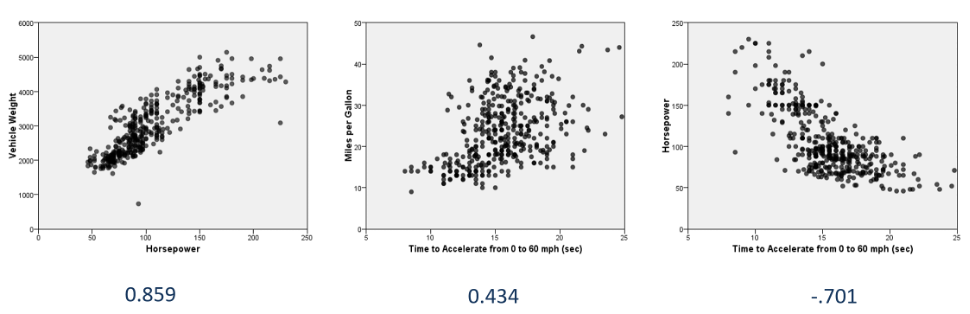# Correlation analysis with SPSS StatisticsIn this webinar Jarlath Quinn takes a deep dive into correlation analysis in SPSS Statistics. Correlations coefficients are one of the most widely-employed analytical techniques used to assess whether linear relationships exist between separate variables. Jarlath looks at how we can use correlations to measure the degree of association between economic factors like price and demand, environmental effects such as temperature and ice cream sales and sociological factors like education levels and employee salaries.

• Why are correlation values useful?
• Interpreting correlation coefficients
• How correlations are calculated
• Automatically highlighting strong correlations
• Linear vs Non-Linear Relationships
• Non-parametric correlations
• Estimating correlation values with Bootstrapping techniques